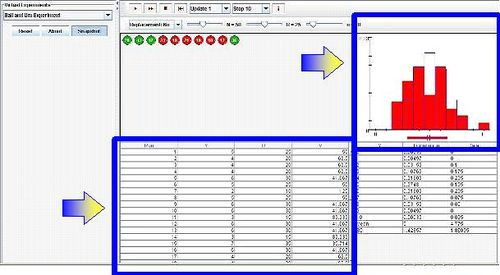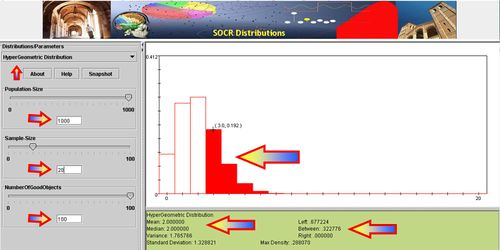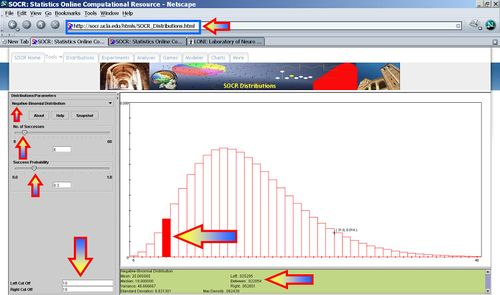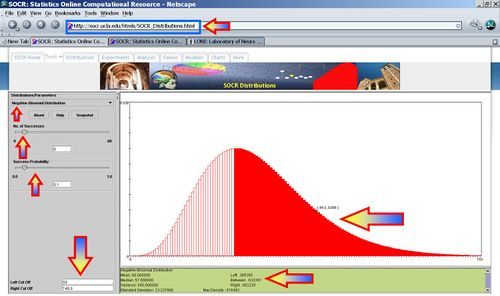# AP Statistics Curriculum 2007 Distrib Dists

## General Advance-Placement (AP) Statistics Curriculum - Geometric, HyperGeometric, Negative Binomial Random Variables and Experiments

### Geometric

• Definition: The geometric distribution is the probability distribution of the number X of Bernoulli trials needed to get one success, supported on the set {1, 2, 3, ...}.
• Mass function: If the probability of successes on each trial is P(success)=p, then the probability that x trials are needed to get one success is $$P(X = x) = (1 - p)^{x-1} \times p$$, for x = 1, 2, 3, 4,....
• Expectation: The expected value of a geometrically distributed random variable X is $${1\over p}.$$
• Variance: The variance is $${1-p\over p^2}.$$

### HyperGeometric

The hypergeometric distribution is a discrete probability distribution that describes the number of successes in a sequence of n draws from a finite population without replacement. An experimental design for using Hypergeometric distribution is illustrated in this table:

 Type Drawn Not-Drawn Total Defective k m-k m Non-Defective n-k N+k-n-m N-m Total n N-n N
• Explanation: Suppose there is a shipment of N objects in which m are defective. The hypergeometric distribution describes the probability that in a sample of n distinctive objects drawn from the shipment exactly k objects are defective.
• Mass function: The random variable X follows the hypergeometric distribution with parameters N, m and n, then the probability of getting exactly k successes is given by

$P(X=k) = {{{m \choose k} {{N-m} \choose {n-k}}}\over {N \choose n}}.$

This formula for the hypergeometric mass function may be interpreted as follows: There are $${{N}\choose{n}}$$ possible samples (without replacement). There are $${{m}\choose{k}}$$ ways to obtain k defective objects and there are $${{N-m}\choose{n-k}}$$ ways to fill out the rest of the sample with non-defective objects.

#### Examples

• SOCR Activity: The SOCR Ball and Urn Experiment provides a hands-on demonstration of the utilization of Hypergeometric distribution in practice. This activity consists of selecting n balls at random from an urn with N balls, R of which are red and the other N - R green. The number of red balls Y in the sample is recorded on each update. The distribution and moments of Y are shown in blue in the distribution graph and are recorded in the distribution table. On each update, the empirical density and moments of Y are shown in red in the distribution graph and are recorded in the distribution table. Either of two sampling models can be selected with the list box: with replacement and without replacement. The parameters N, R, and n can be varied with scroll bars.• A lake contains 1,000 fish; 100 are randomly caught and tagged. Suppose that later we catch 20 fish. Use SOCR Hypergeometric Distribution to:
• Compute the probability mass function of the number of tagged fish in the sample of 20.
• Compute the expected value and the variance of the number of tagged fish in this sample.
• Compute the probability that this random sample contains more than 3 tagged fish.### Negative Binomial

• Definition: The family of negative binomial distributions is a two-parameter family; p and r with 0 < p < 1 and r > 0.
• Mass Function: The probability mass function of a Negative Binomial random variable (X~NegBin(r, p))is give by:

$P(X=k) = {k+r-1 \choose k}\cdot p^r \cdot (1-p)^k \!$, for k = 0,1,2,....

#### Application

Suppose Jane is promoting and fund-raising for a presidential candidate. She wants to visit all 50 states and she's pledged to get all electoral votes of 6 states before she and the candidate she represents are satisfied. In every state, there is a 30% chance that Jane will be able to secure all electoral votes and 70% chance that she'll fail.

• What's the probability mass function that the last 6th state where she succeeds to secure all electoral votes happens to be the at the nth state she campaigns in?

NegBin(r, p) distribution describes the probability of k failures and r successes in n=k+r Bernoulli(p) trials with success on the last trial. Looking to secure the electoral votes for 6 states means Jane needs to get 6 successes before she (and her candidate) is happy. The number of trials (i.e., states visited) needed is n=k+6. The random variable we are interested in is X={number of states visited to achieve 6 successes (secure all electoral votes within these states)}. So, k = n-6, and $$X\sim NegBin(6, 0.3)$$. Thus, for $$n \geq 6$$, the mass function (giving the probabilities that Jane will visit n states before her ultimate success is:

$P(X=n) = {(n-6) + 6 - 1 \choose 6-1} \; 0.3^6 \; 0.7^{n-6} = {n-1 \choose 5} \; \frac{3^6 \; 7^{n-6} \; }{10^n}$

• What's the probability that Jane finishes her campaign in the 10th state?

$P(X=10) = 0.022054$• What's the probability that Jane finishes campaigning on or before reaching the 8th state?

$P(X\leq 8) = 0.011292$

Error creating thumbnail: File missing
• Suppose the success of getting all electoral votes within a state is reduced to only 10%, then X~NegBin(r=6, p=0.1). Notice that the shape and domain the Negative-Binomial distribution significantly chance now (see image below)! What's the probability that Jane covers all 50 states but fails to get all electoral votes in any 6 states (as she had hoped for)?

$P(X\geq 50) = 0.632391$